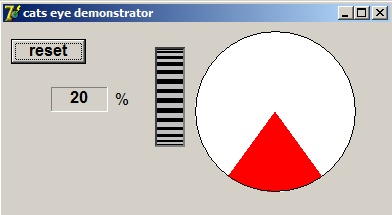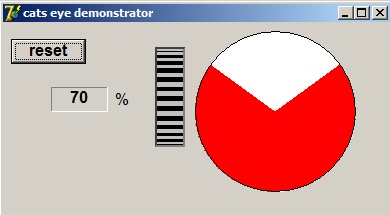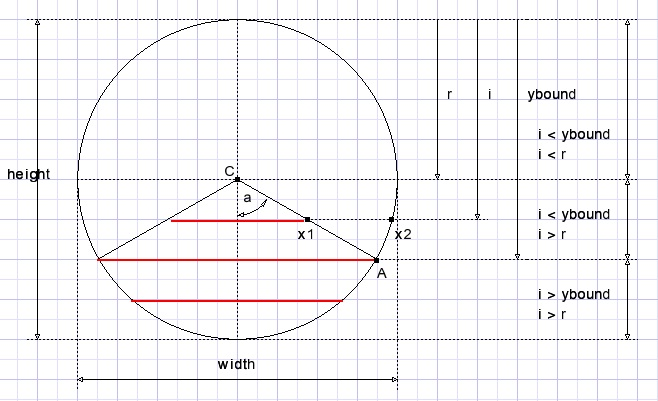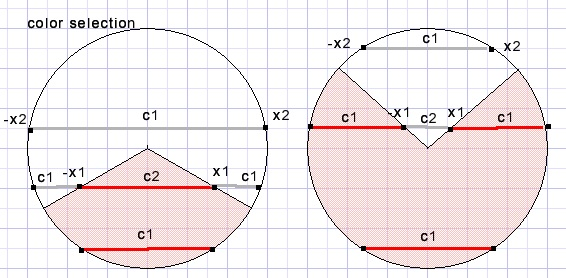Programming a cat's eye indicatordownload cat's eye programdownload cat's eye Delphi project

A cat's eye indicator

also called magic eye, is a fluorescent cathode ray indicator used for radio receiver tuning.A voltage controls the size of illumination. This Delphi project paints a cat's eye indicator to be used for progress reporting.

Picure below shows the project at work:Painting is done on a TImage component.
A rotation button (home brew component) supplies a number 0..100 as percentage to the cat's eye paint procedure.
The reset button causes the TImage to set it's canvas to the form color.
Drawing of the cat's eye does not alter pixels outside the circle.

AnalysesVariable i steps from height-1 downto 0.
Painting is done by drawing horizontal lines for each i.
Center C has coordinates (0,0) during calculations.
Radius r := height div 2.
Angle a (radians) depends on the percentage v.
a := 0.01*v*pi {pi = 180 degrees}
ta := tan(a) the slope of line CA.
Vertical coordinate of A is ybound := round(r*(1+cos(a)))
x1,x2 are calculated for each i.
x2 := round(sqrt(r2 - sqr(i-r)))..........on circle
x1 := round((i-r)*ta).....................on lineThe following cases for line drawing are observed:
1. red : -x2 to x2
2. white: -x2 to -x1, red: -x1 to x1, white: x1 to x2
3. red: -x2 to -x1, white -x1 to x1,red: x1 to x2
4. white: -x2 to x2To distinguish between these cases 2 flags (Boolean variables) are used:
BF := i > ybound
RF := i > r
Colors c1,c2 are set to red or white according to BF,RF
Then procedure line(xstart,xend,color ) is called to draw the lines at y = i
Also the line procedure adds r to the x coordinates

Finally the black circle is drawn.
Three lines are required if (BF xor RF) is true.
In the other cases one line is sufficient.
Please refer to the source code for more details.

Image dimensions

Different image dimensions may be selected.
Make sure that width = height.
Best is to make width and height odd, so center C is really the center of the circle.

The cat's eye paint procedure

```procedure paintCatsEye(v : byte);
//v is percentage 0..100
var i,r,r2,x1,x2,ybound : smallInt;
a,ta : single;
BF,RF : boolean;
c1,c2 : dword;   //colors

procedure line(a,b: smallInt; col : dword);
begin
with form1.Image1.Canvas do
begin
pen.Color := col;
moveto(a+r,i);
lineto(b+r,i);
end;
end;

begin
with form1.Image1 do
begin
r := width shr 1;
r2 := r*r;
a := 0.01*v*pi;   //angle in radians
if v = 50 then ta := 10000 else ta := tan(a);
ybound := round(r*(1+cos(a)));
for i := height-1 downto 0 do
begin
BF := i > ybound;
RF := i > r;
x2 := round(sqrt(r2 - sqr(i-r)));
if BF then begin
c1 := \$ff;
c2 := \$ffffff;
end
else begin
c1 := \$ffffff;
c2 := \$ff;
end;
if RF xor BF then
begin
x1 := round((i-r)*ta);
line(-x2,-x1,c1);
line(-x1,x1,c2);
line(x1,x2,c1);
end
else line(-x2,x2,c1);
end;//for i
with canvas do
begin
brush.style := bsClear;
pen.color := 0;
ellipse(0,0,width,height);
end;
end;//with
end;
```

The procedure to initialize the image

```procedure InitCatsEye;
begin
with form1.Image1 do with canvas do
begin
brush.style := bsSolid;
brush.Color := form1.Canvas.Brush.color;
fillrect(rect(0,0,width,height));
end;
end;
```
This concludes the cat's eye paint description.

The rotation button description and source code may be found [HERE]